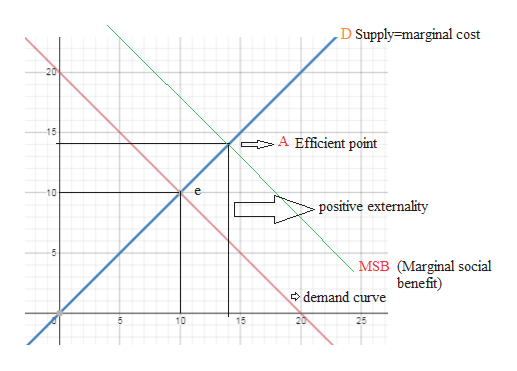# Suppose demand isUpper Q Subscript Upper D equals 20 minus Upper PQD = 20−Pand supply isUpper Q Subscript Upper S Baseline equals Upper PQS=P.There is a constant positive externality of​\$4per unit​ (Marginal External​ Benefit,MEB equals \$ 4MEB =\$4​).The adjacent graph shows the supply curve described above. On this graph illustrate the demand curve and marginal social benefit curve and find the socially efficient point. ​1.) Use the line drawing tool to illustrate the demand curve given above. Label this curve​ 'D'. ​2.) Use the line drawing tool to illustrate the marginal social benefit curve given the information above. Label this curve​ 'MSB'. ​3.) Use the point drawing tool to illustrate the socially efficient price and quantity. Label this point​ 'A'. Carefully follow the instructions​ above, and only draw the requiredobjects.

Question
177 views
Suppose demand is
Upper Q Subscript Upper D equals 20 minus Upper PQD = 20−P
and supply is
Upper Q Subscript Upper S Baseline equals Upper PQS=P.
There is a constant positive externality of
​\$4
per unit​ (Marginal External​ Benefit,
MEB equals \$ 4MEB =\$4​).
The adjacent graph shows the supply curve described above. On this graph illustrate the demand curve and marginal social benefit curve and find the socially efficient point.

​1.) Use the line drawing tool to illustrate the demand curve given above. Label this curve​ 'D'.

​2.) Use the line drawing tool to illustrate the marginal social benefit curve given the information above. Label this curve​ 'MSB'.

​3.) Use the point drawing tool to illustrate the socially efficient price and quantity. Label this point​ 'A'.

Carefully follow the instructions​ above, and only draw the required
objects.
check_circle

Step 1

The positive externality shows the benefits to the third party during any economical transaction. When there is positive externality in the economy then the marginal social benefit is greater than the marginal private benefits.

Step 2

Given,

Demand function Qd = 20-p

Supply function QS = P

Constant positive externality = \$4

Marginal external benefi...help_outlineImage TranscriptioncloseD Supply marginal cost 20 15 A Efficient point e -10 positive externality P MSB (Marginal social benefit) demand curve 20 5 10 15 25 fullscreen

### Want to see the full answer?

See Solution

#### Want to see this answer and more?

Solutions are written by subject experts who are available 24/7. Questions are typically answered within 1 hour.*

See Solution
*Response times may vary by subject and question.
Tagged in